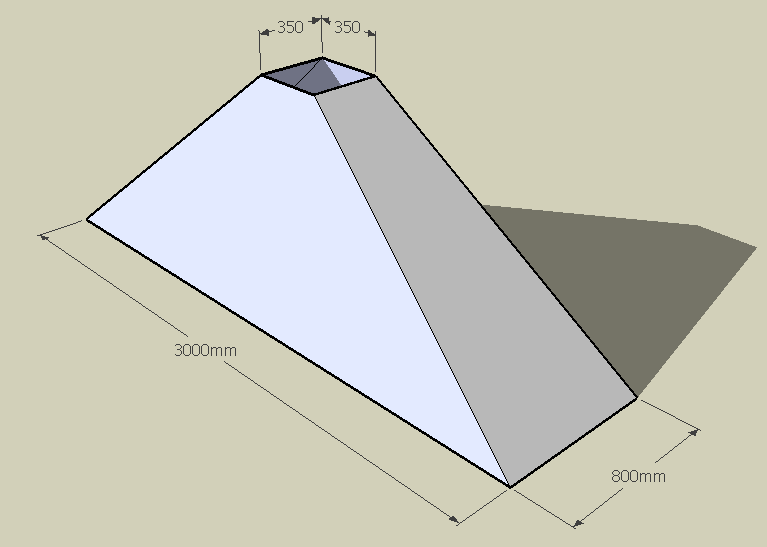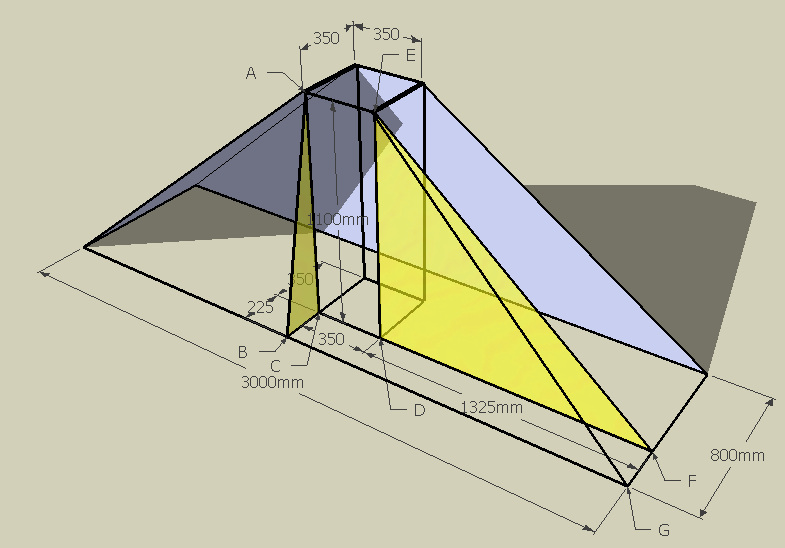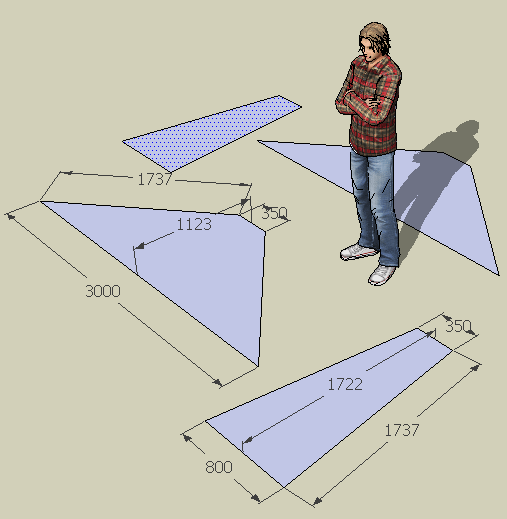SEARCH HOMEMath Central Quandaries & QueriesQuestion from phillip: Hi, I have a rectangular base to square top, pyramid shape to fabricate out of steel plate (4 sections). The base is 3000mm by 800mm, the height is 1100mm and the top is 350mm square. What I need to know is the lengths of the sides of the plate(were they meet vertically). Hope ive made it clear enough. Thanks Phil.Hi Phil.

Is the sketch below what you are making?Let me cut away the solid sides and show you some of the useful internal triangles that will solve this problem:We can solve your question by using the Pythagoras' Theorem (a2 + b2 = h2). It relates the lengths of the sides of a right triangle to the length of its hypotenuse.

First consider triangle ABC. We know the length of AC is the height (1100) and the length of BC is 225
( (800-350)/2 ). So AB is the hypotenuse and it is sqrt(11002 + 2252) which is about 1123 mm. That is the "slant height" of the large pieces.

Now look at triangle DEF. We know ED is 1100 and DF is 1325 ( (3000 - 350) / 2 ). That makes the slant height of the narrow pieces (EF) equal to sqrt(13252 + 11002) which is about 1722 mm.

Finally, look at the triangle EFG. We know FG = 225 and (now) we know EF is 1722. So EG is sqrt(2252 + 17222) which is about 1737 mm.

Now we have all the measurements we need. Here are the two patterns we use for the four pieces:Hope this helps,
Stephen La Rocque.Math Central is supported by the University of Regina and The Pacific Institute for the Mathematical Sciences.